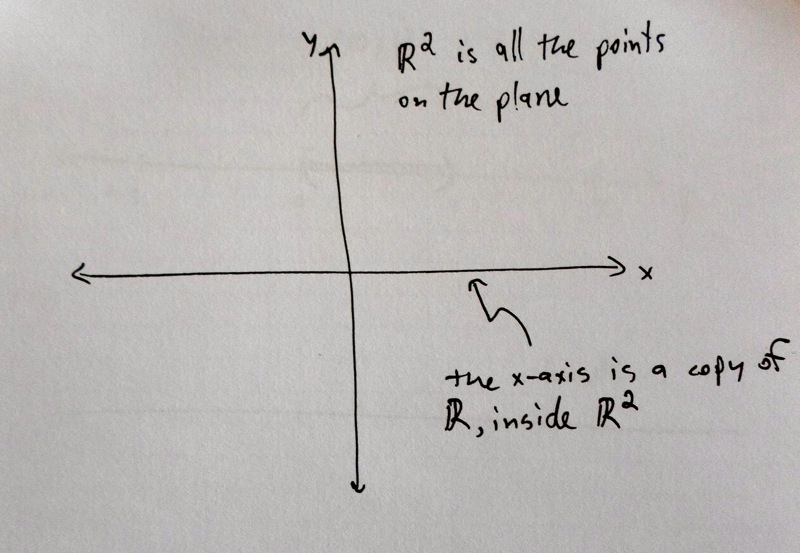# Short

Here is a rough sketch of why $$|\mathbb{R}^4| = |\mathbb{R}|$$.

Actually, we'll explain something slightly simpler: that $$|\mathbb{R}^2| = |\mathbb{R}|$$. From this simpler explanation, the argument for the more complicated case will be apparent, and likewise the argument for why $$|\mathbb{R}^n| = |\mathbb{R}|$$ for any positive number $$n$$.

Rather than construct a one-to-one correspondence between $$\mathbb{R}^2$$ and $$\mathbb{R}$$ directly, we're going to use a different technique.

Remember that $$\mathbb{R}$$ is the continuous one-dimensional real line, and $$\mathbb{R}^2$$ is the continuous two-dimensional plane. We can imagine a copy of $$\mathbb{R}$$ sitting inside $$\mathbb{R}^2$$, as the $$x$$-axis of a coordinate system. The elements of $$\mathbb{R}^2$$ are of the form $$[x, y]$$, where $$x$$ and $$y$$ are real numbers. Then the $$x$$-axis is all the points of the form $$[x, 0]$$, where $$x$$ is any real number. (The $$x$$-axis is exactly the points where the $$y$$-coordinate is zero.)So we can construct a one-to-one correspondence between $$\mathbb{R}$$ and the $$x$$-axis of the plane. Every real number $$x$$ gets assigned to $$[x, 0]$$. This clearly hits every point on the $$x$$-axis once and only once. Since the $$x$$-axis is a subset of the whole plane $$\mathbb{R}^2$$, we can conclude that $$|\mathbb{R}| ≤ |\mathbb{R}^2|$$.

So far this isn't so surprising. But next we'll explain that $$|\mathbb{R}^2| ≤ |\mathbb{R}|$$.

Every element of $$\mathbb{R}^2$$ looks like $$[x, y]$$, for some real numbers $$x$$ and $$y$$. Make a rule that assigns each such pair to one real number, by interweaving the digits of $$x$$ and $$y$$. A few examples will give the idea.

$$[0.111111…, 0.2222222…]$$ gets assigned to $$0.1212121212…$$.

$$[13, 200]$$ gets assigned to $$21030$$.

$$[485.2, 3.778]$$ gets assigned to $$408053.270708$$.

To do this carefully, we have to line up the digits of $$x$$ and $$y$$ so they have the same number of digits, and their decimal points match. This isn't a problem, since we can always add zeros to the left and right as needed. So we think of $$[13, 200]$$ as $$[013.0, 200.0]$$, and this gets assigned to $$021030$$.

This rule assigns every element of $$\mathbb{R}^2$$ to an element of $$\mathbb{R}$$. It turns out that it doesn't actually hit every element of $$\mathbb{R}$$ (read the In-Depth explanation to see why).

But the good news is that every element of $$\mathbb{R}$$ it does hit, it only hits once. Another way of thinking of this is that we can undo the rule. From a real number like $$0.121212…$$, it's possible to recover the point $$[0.111…, 0.222…]$$ that hit it. Just unweave the real number into two.

Because it is possible to unweave, we can conclude that our assignment rule makes a one-to-one correspondence between $$\mathbb{R}^2$$ and a subset of $$\mathbb{R}$$ (not all of $$\mathbb{R}$$, because it doesn't hit everything). And this is enough to conclude that $$|\mathbb{R}^2| ≤ |\mathbb{R}|$$.

It turns out that infinities work like regular numbers, in the sense that $$a ≤ b$$ and $$b ≤ a$$ means that $$a = b$$. (This is actually a hard theorem to prove.)

So, since we have $$|\mathbb{R}| ≤ |\mathbb{R}^2|$$ and $$|\mathbb{R}^2| ≤ |\mathbb{R}|$$, we can conclude that $$|\mathbb{R}^2| = |\mathbb{R}|$$.

Top